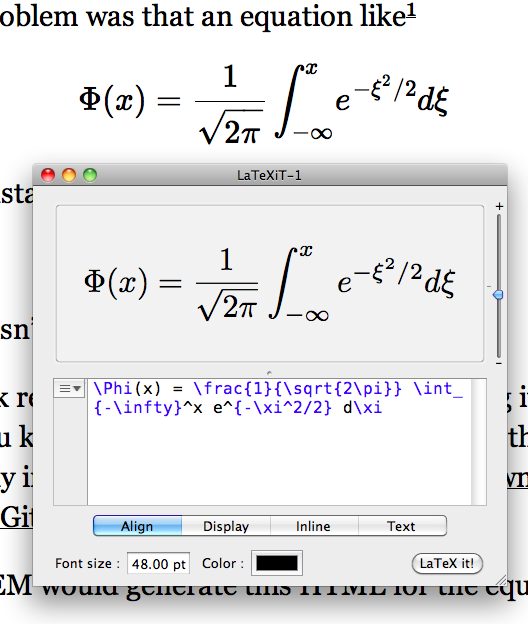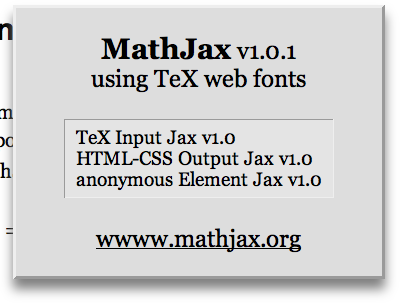# A small request

I’ve been having a discussion on the MathJax mailing list about which fonts get used to render equations. According to the MathJax font help page, the STIX fonts are supposed to be used if you have them on your local computer. I do have the STIX fonts installed on both my iMac and my Macbook Air, but on both computers the equations are rendered in the “TeX” font, which is an Open Type version of Don Knuth’s Computer Modern font.

Here’s a screenshot showing one of my posts (with the font size greatly increased) in the background and LaTeXiT rendering the same formula in Computer Modern in the foreground.Apart from a small difference in weight, these are clearly the same font. For some reason, my computers are refusing to use the STIX fonts to render the equations.

An easier way to see which fonts are being used to render the equations is to right-click on an equation and choose “About MathJax” from the popup menu. You’ll probably see this,which shows that your system is using the TeX fonts from my server.

Here’s my request: Could some of you install the STIX fonts on your computers and tell me the font being used in the equations on your machine? You can use this equation for the test:

$\Phi(x) = \frac{1}{\sqrt{2\pi}} \int_{-\infty}^x e^{-\xi^2/2} d\xi$

I’d like to know whether the problem I’m having is widespread or something unique to my setup. If it’s widespread, I think the MathJax people should know about it.